Create a new printableGeometry
Math Worksheets

Sample - Click above to make a new math worksheet (PDF).
 Name _____________________________Date ___________________
Area of a Circle
To the nearest tenth, find the area of each circle. Use 3.14 for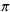.

1.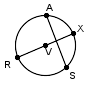RX
=  4.5 ft
2.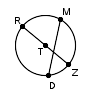ZT
=  10.285 mm
3.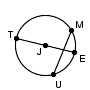JT
=  31.7 cm
4.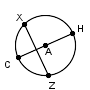CH
=  14.5 in
5.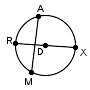RD
=  36.77 mm
6.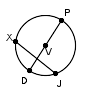VP
=  3.314 ft
7.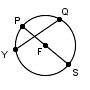SP
=  42.2 cm
8.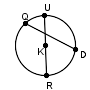UR
=  15.26 in
9.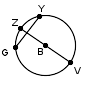ZV
=  12.328 in
10.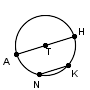HA
=  22.918 ft
 11 * This is a pre-made sheet.Use the link at the top of the page for a printable page.
12.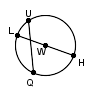HW
=  33.8 mm
13.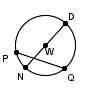ND
=  13.212 mm
14.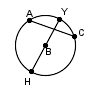BH
=  16.9 ft